Graphing Linear Inequalities
 Example 1:    Graph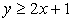Enter 2x + 1 into Y1 Arrow to the far left side of Y1 Hit ENTER until the "shade above" symbol is displayed. Hit ZOOM #6 ZStandard (for a 10x10 window) Graph NOTE:  You will have to determine whether to draw a solid line or a dotted line for  y = 2x + 1.  This problem uses a solid line because of the "less than or equal to" sign.  The calculator will display a solid line at all times. (The Application program called "Inequalities" will display solid and dotted lines on the graphs.)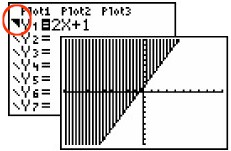Example 2:    Graph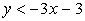Notice the "shade below" symbol to the far left of the Y1. Again, you must determine whether to draw a solid or dotted line.  This problem uses a dotted line because it is strictly "less than" (no "equal to").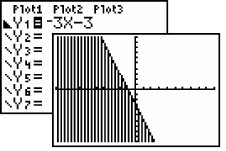Example 3:   Solve the system: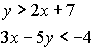You will need to isolate the y variable in the second equation so that it can be entered into the calculator with the "shade above" indicated.        Solving algebraically,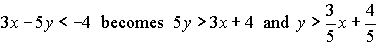(be careful of the direction of the inequality symbol in this problem.) The answer is the double shaded region on the graph.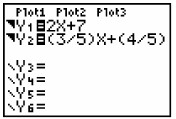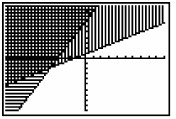Example 4:        Solve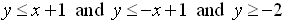Enter all three equations with appropriate "shade above" or "shade below" symbol. Answer is the darkest shading.Zoom Decimal view.